﻿ Energy
Home | Previous | Up one level | Next

#### § 4. -- ENERGY WALTER RITZ

##### Translated (1980) from Recherches critiques sur l'Électrodynamique Générale, Annales de Chimie et de Physique, Vol. 13,   p. 145, 1908.

Annales 172 (Oeuvres 340)

Maxwell has shown, in all the particular cases with which we are acquainted regarding electromagnetic energy, that the energy can be expressed in the form of an integral extended over all space, which in Lorentz's hypothesis takes the formMaxwell admits, and this is an important point in his system, that each element of volume is effectively, and in all cases, the seat of a quantity of energy equal toPoynting has since shown that this results from the general equations, that for all closed surfaces σ we have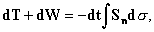where dT is the work of outside forces, andis the surface component in the direction of the normal external radial vector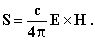The theorem states itself elegantly in considering the energy as being comprised of an indestructible fluid which moves parallel to the radiant vector, an image which presents certain advantages but lays itself open to much criticism.(1) Particularly, we can ask ourselves if the statement "continuous energy in a given volume with such and such value," has any real sense when we can only define differences of energy.
We will set aside this metaphysical question to study the indetermination that this mode of representation suffers. (Oeuvres 341) The electrostatic energy of a system is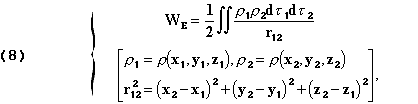the two integrations being extended over all space wherever electric charges are found.
It is evident that this expression can be put in the form of an extended integral throughout all space in an infinite number of ways and the one Maxwell has chosen is, from the point of view of his system, particularly simple. But we can show others which will have for example,

##### (1)See W. Wein, Annalen der Physik u. Chemie, 2nd series, volume XLV, 1892,   p. 684.

the advantage of drawing closer to the forms used in the theory of elastic bodies. Lets therefore introduce, instead of the electric force E in x,y,z which is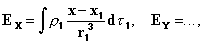the vectorfor which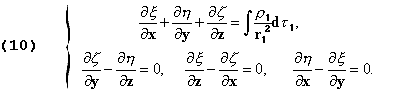Now, if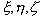were the components of displacements for an elastic body, the energy of this latter vector would be, as we knowλ and μ being constants of elasticity of the body, or, in adding the
(Oeuvres 342) zero terms, . . . and transforming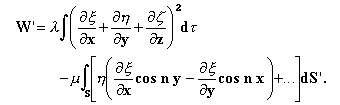If the elastic body is infinitely extended, by virtue of formula (9), the surface integral will vanish and leave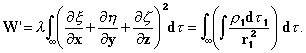For electricity, supposedly being firmly attached to small solid bodies (ions)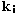, we have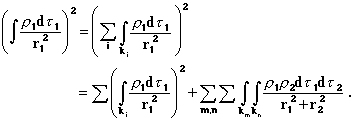The first term doesn't depend on the mutual positions of the bodies. Its integral with regard to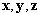extended over all space will be a constant. In the double summation the variables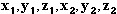assume the gamut of relative values for a given combination of different ions. We therefore never have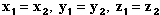which allows the transposition of the order of integration and we write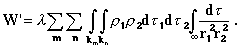To evaluate the integral taken with respect tolet's introduce polar coordinateswithas the polar origin, the line joiningand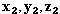as the polar axis. Let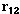be the distance between these two points. We have to calculate(Oeuvres 343)

The principal values (included betweenand) are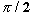for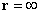, and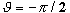for.   At last we findfrom whichWe conclude finally, in choosing λ = 1/2π3, that with the hypotheses made and with the units introduced, the elastic energy W' will, having a nearly constant summation, be equal to the electrical energy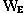given by (8), and that we have in particular the formula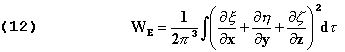which is irreducible to Maxwell's formula, and gives a completely different distribution for the energy. It would be easy to obtain, by starting in this manner, a similar expression for magnetic energy and, consequently, for currents; and we see that, even in insisting on the simplest of formulas, the localization of energy is still an indeterminant problem.
It is the same for the flux of energy.(1) We can always modify the motion of the flowing energy in an arbitrary way by adding to Poynting's vector another vector (u,v,w) obliged only to satisfy the equation for incompressible fluids.from which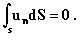Poynting's theorem, being a consequence of the general equations, doesn't add anything to them. The localization of energy

* This is the second equation numbered "11."

##### (1) See the article of M. Voss in Encyklop. d. math. Wissenschaften, vol. IV, art. 1, 1901, p. 111-114.

must therefore be attributed to a number of logically useless (and maybe harmful at times) conceptions in the theory.

(Oeuvres 344)
But there is another aspect for which it's important to consider Poynting's theorem.
The primary source of the conservation of energy theorem has been, and will continue to be, the experimental impossibility of perpetual motion, an impossibility that must exist whatever our ideas may be on the portions of energy that the ether is obliged to supply in the absence of material bodies. The energy theorem, in its classical form

W=Const.

explained this impossibility. Poynting's theorem, in demanding only the possibility of the transformation of an integral of volume (already partly arbitrary) to an integral of surface, expresses a lot less. Far from giving an account for this impossibility, it readily allows the creation of a perpetual motion. This is to say that, as long as we have not introduced the hypothesis of retarded potentials, a continuous portion of the energy, from converging waves coming from infinity, remains just as possible as the lost energy that we observe in reality. If an engine could perpetually draw energy from ether solely, independently of the presence of material bodies, it could have perpetual motion. We know therefore, that in adopting the formula for retarded potentials, we must show(1) that an accelerated particle loses energy and undergoes, as a result, a reaction proportional to the derivative of its acceleration. We only have to change the sign of c to pass to the hypothesis of convergent waves. We see then that the sign of the radiant vector changes also, and

##### (1) LORENTZ, Elektronentheorie, p. 186 - LARMOR, Aether and Matter, Chap. XIV.

the new hypothesis will lead, for example in the case of a vibrating particle, to a continuous increase in the amplitude with time, and more generally to an increase of energy of the system.
Poynting's theorem expresses the law of energy only when we replace the fields with their representations based on retarded potentials, a restriction which removes a lot of its elegance and scope.
If we begin from a state in which radiation is sensibly nil and where the energy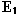can be converted into work, and we arrive at an (Oeuvres 345) analogous state (energy), the system supposedly isolated from the action of external bodies, we will have, according to Lorentz's theory, (which supposes the admissibility of the formula for potentials)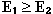the equality taking place only if radiation is continuously nil. The impossibility of perpetual motion also produces, in an essentially irreversible system, only the inequality. The energy can never increase. There is a parallelism in this relation with the law of entropy. In fact, electromagnetic energy is not conserved in general. This is to say that W= const. doesn't exist. We save the law of conservation of energy by attributing to the ether the lost quantity, and this procedure has decidedly great advantages, especially when we can completely recover the energy lost by the system by means of bodies that don't sensibly exert action on it, like the black bodies of optics. But with this energy not producing, in this hypothetical setting, any modifications which would be perceptible to our senses, we can ask ourselves if, under these conditions, would it not be possible to save likewise all other similar laws, as we have effectively done for electromagnetic momentum.

In the most general case of electromagnetic radiation, conservation of energy is no longer a law, but a convention. This is a fairly frequent development in the domain of physical truths, as stated by Poincaré(1).